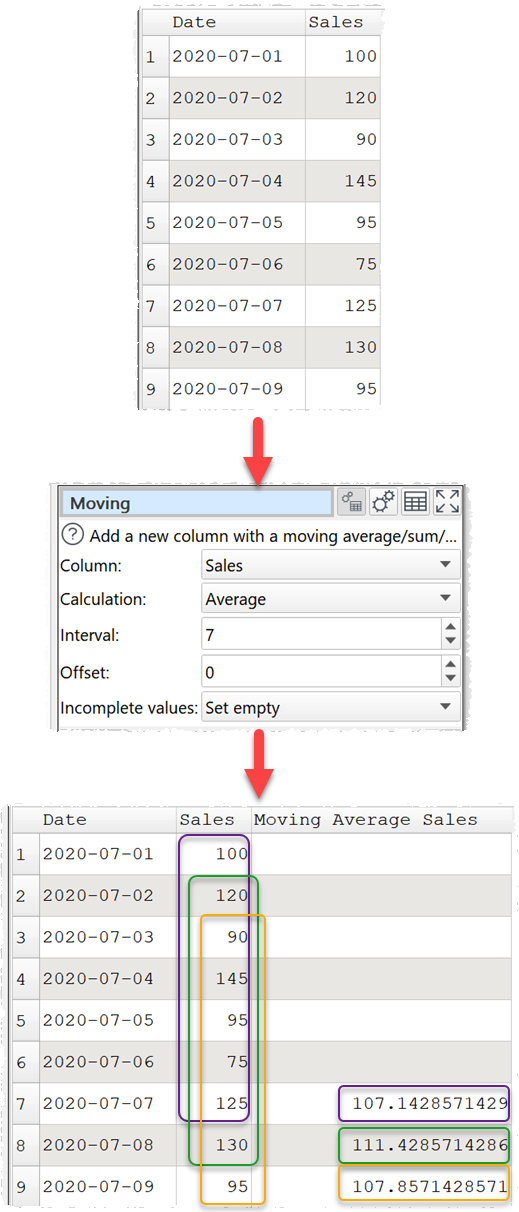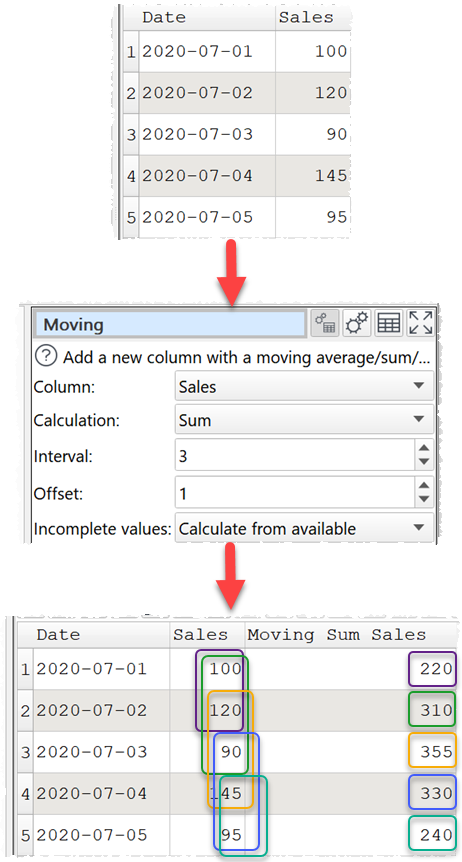﻿ Reference > Transforms > Moving

# Moving

## Examples

Calculate a 7 day moving average for the sales column, leaving the first 6 days empty.Calculate the sum of the current row and the rows above and below, where available, for the sales column.One.

## Options

Select the Column you wish to calculate a moving average/median/sum/minimum/maximum for.

Set Calculation to the statistic to be calculated.

oAverage shows the arithmetic mean of the values in the interval (Simple Moving Average).

oMedian shows the median of the values in the interval.

oSum show the sum of the values in the interval.

oMinimum shows the smallest value in the interval.

oMaximum shows the largest value in the interval.

Set Interval to the number of rows to include in each moving average/median/sum/minimum/maximum value.

Set Offset to the number of rows above (if negative) or below (if positive) the current row to end each interval at.

Set Incomplete values according to what you want to show for rows without a full interval of data. For example the first 6 rows when Interval is 7 and Offset is 0.

## Notes

Non-numeric values are ignored. E.g. a 7 day moving average that includes 2 non-numeric values will be shown as an average of the 5 numeric values.

You will be warned of any non-numerical values in the selected column.